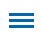Completing computations is a straightforward process for intermediate-ages students and can be made more meaningful by connecting to an authentic, real life scenario.

In Wixie's Orbits and Leap Years template, students are required to reflect on Earth's orbit and the need for a Leap Year, as well as calculate age based on the orbits of different planets. The template also leaves plenty of options to expand the task to better challenge students based on their computation skills.

Editor's Note: This blog is part of a series of posts from former math specialist Scott Loomis on the real world math templates he has created for Wixie and how you can use and modify them for your own awesome performance tasks. You can read the backstory on his experience and expertise below.

## Orbits and Leap Years

template outlines the orbit of Earth around the sun and the use of the Leap Year. That need for a Leap Year is outlined, mathematically, on page 3 and the students are asked to reflect on what might happen if the Leap Year was not part of our calendar. The final two pages ask students to calculate the number of "years" old they would be on different planets - Venus, Mercury, and Mars. Completing long division involving two- and three-digit dividends and divisors is required.

Wixie makes it easy to open and customize existing templates. We encourage you to modify* the existing problems and activities to meet your specific computation expectations.

Instructional Concept: Perform operations with multi-digit whole numbers and with decimals to hundredths.Location in Templates Library:  Curriculum > Math  > Numbers and Operations > Computation > Multiplication and Division

Estimated Completion Time: 10-20 minutes

## Math Standards and Learning Goals

### Common Core State Standards  Grade 5 Mathematics - Number & Operations in Base Ten

5.NBT.B.6. Find whole-number quotients of whole numbers with up to four-digit dividends and two-digit divisors, using strategies based on place value, the properties of operations, and/or the relationship between multiplication and division. Illustrate and explain the calculation by using equations, rectangular arrays, and/or area models.

### Grade 6 Mathematics - The Number System

6.NS.B.2. Fluently divide multi-digit numbers using the standard algorithm.

### Virginia Standards of Learning - Mathematics - Computation & Estimation

5.4 The student will create and solve single-step and multistep practical problems involving addition, subtraction, multiplication, and division of whole numbers.

### Grade 5 - Number & Operations

TX.5.NO.3. The student applies mathematical process standards to develop and use strategies and methods for positive rational number computations in order to solve problems with efficiency and accuracy. Student is expected to:

(C) solve with proficiency for quotients of up to a four-digit dividend by a two-digit divisor using strategies and the standard algorithm.

### Grade 6 - Number & Operations

TX.6.NO.3. The student applies mathematical process standards to represent addition, subtraction, multiplication, and division while solving problems and justifying solutions. The student is expected to:

(E) multiply and divide positive rational numbers fluently.

## Wixie Skills Review

Most of the work on this template will be completed using the paintbrush tool. It is helpful to set it to its smallest size so it can be used as a pencil.The third page requires the insertion of an audio recording. Teachers are encouraged to review this process using the Record, Pause, and Stop options and the Try Again and Keep. Audio recording can last up to five minutes in length, and the use of a script is discouraged. Instead, permit the students to "ramble" about the process they used to multiply and divide as well as any problem-solving strategies they may have utilized.*If you are modifying the template to better match the needs and expectations of your learners, you can edit the template. In this file, the objects on the page are locked, so you will need to choose Unlock on the toolbar to make changes. This option can also be found under the Edit menu and Properties. To learn more, visit the Creating custom curriculum in Wixie playlist on YouTube.

Topics: Wixie, math, real world of math